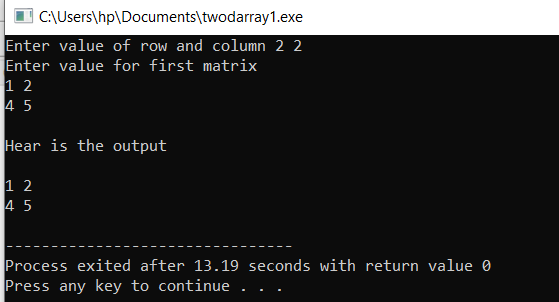### Ticker

20/recent/ticker-posts

# Two dimensional array in c++ - Algomentor

## A Two dimensional array in c++ with solved example

Welcome friend in this article we will learn about a two dimensional array in c++. you can imagine a two dimensional array in c++ as a matrix you study in Mathematics. or you can understand as a grid of row and columns or you can visualize a two dimensional array as a classroom full of students in which student sit in benches these benches is a row, and there are multiple benches in the classroom they form a structure like a two dimensional grid in which student sit in rows and columns.

Note: If you don't know about an array I recommend you go through our array tutorial. because a one-dimensional array is essential to learn about two dimensional array.

What is the two dimensional array in c++?

A two dimensional array can say as an array of an array in which multiple arrays stores together of the same type. The relational database of MySql in the form of a table and tables are two dimensional array.

Here is the pictorial representation of a two dimensional array in c++ or any other programming language two dimensional array are the same.

So you can see that the two dimensional array looks like a grid which consists of rows and columns in some time we will learn about practical uses and how to make two dimensional array coding question. so be with us for some time 😀.

What are some practical use cases of a two dimensional array in c++?

• Use in a relational database to stores user data in the form of tables.
• To the solved problem of graphs (A non-linear data structure)
• To solve the problem of traveling salesmen in minimum coast
• we can make a map of the country/state with city distance and can store in two dimensional array.
So you can see there are many practical use cases of the two dimensional array so much should have a good inside of two-dimensional array so that we can solve the coding problem as well as many complex problems with the concept of a two-dimensional array.

What are the different ways to initialize a two dimensional array in c++?

• int arr;
• int arr = {1,2,3,4,5,6,7,8,9,10,11,12};
• int arr = {{1,2,3,4},{5,6,7,8},{9,10,11,12}};
Their is the pictorial represenation of array of size 3*4 in with element {1,2,3,4,5,6,7,8,9,10,11,12} in the grid.

So now you can easily visualize how we store elements in two dimensional array {1,2,3,4} in first row {5,6,7,8} in second row {9,10,11,12} in third row.

Write A program to take input of two-dimensional array and print it on screen?

#include<iostream>
using namespace std;
int main()
{
int row,column;
cout<<"Enter value of row and column ";
cin>>row>>column;
int arr[row][column];
cout<<"Enter value for first matrix "<<endl;
for(int i=0;i<row;i++)
{
for(int j=0;j<column;j++)
{
cin>>arr[i][j];
}
}
cout<<endl<<"Hear is the output"<<endl<<endl;
for(int i=0;i<row;i++)
{
for(int j=0;j<column;j++)
{
cout<<arr[i][j]<<" ";
}
cout<<endl;
}
}

The output of the codeTake a Matrix of 3*3 from the user and print its determinant value?

#include<iostream>
using namespace std;
int main()
{
int row,column;
int arr;
cout<<"Enter value for first matrix "<<endl;
for(int i=0;i<3;i++)
{
for(int j=0;j<3;j++)
{
cin>>arr[i][j];
}
}
int val1 = arr*((arr*arr)-(arr*arr));
int val2 = arr*((arr*arr)-(arr*arr));
int val3 = arr*((arr*arr)-(arr*arr));
int valOfDeterminant = val1-val2+val3;
cout<<"Hear is the value of above determinant "<<valOfDeterminant;
}

The output of the code

Write A program to take 2 matrices from the user and print the sum of the matrix?

#include<iostream>
using namespace std;
int main()
{
int row,column;
cout<<"Enter value of row and column ";
cin>>row>>column;
int arr1[row][column];
int arr2[row][column];
cout<<"Enter value for first matrix "<<endl;
for(int i=0;i<row;i++)
{
for(int j=0;j<column;j++)
{
cin>>arr1[i][j];
}
}
cout<<"Enter value for second matrix "<<endl;
for(int i=0;i<row;i++)
{
for(int j=0;j<column;j++)
{
cin>>arr2[i][j];
}
}
cout<<endl<<"Hear is the sum of matrix "<<endl<<endl;
for(int i=0;i<row;i++)
{
for(int j=0;j<column;j++)
{
cout<<arr1[i][j]+arr2[i][j]<<" ";
}
cout<<endl;
}
}

The output of the code

Write A program to take 2 matrices from the user and print the multiplication of the matrix?

#include<iostream>
using namespace std;
int main()
{
int row;
cout<<"Enter value of row: ";
cin>>row;
int arr1[row][row];
int arr2[row][row];
int res[row][row];
cout<<"Enter value for first matrix "<<endl;
for(int i=0;i<row;i++)
{
for(int j=0;j<row;j++)
{
cin>>arr1[i][j];
}
}
cout<<"Enter value for second matrix "<<endl;
for(int i=0;i<row;i++)
{
for(int j=0;j<row;j++)
{
cin>>arr2[i][j];
}
}
for (int i=0; i<row; i++)
{
for (int j=0; j<row; j++)
{
res[i][j] = 0;
for (int k=0; k<row; k++)
{
res[i][j] += arr1[i][k]*arr2[k][j];
}
}
}
cout<<endl<<"Here is matrix multiplication "<<endl<<endl;
for(int i=0;i<row;i++)
{
for(int j=0;j<row;j++)
{
cout<<res[i][j]<<" ";
}
cout<<endl;
}
}

The output of the code

Hope you like our article on a two dimensional array in c++. then please share this article with your coder friends and do follow us on our social or you have any problem or want to give suggestions then, please go on the main page and you find a contact form where you can mention your problem/suggestion in the message box.